# Normal Distribution Definition

  

The probability density function of the normal distribution and its properties are presented starting from the probability histograms . A normally distributed data set with mean $$\mu = 3.5$$ and a standard deviation $$\sigma = 1$$ is used to highlight the link between the probability histogram of the data and the normal density function which leads to the definition of normal distribution .
Graphs of normal distributions are presented to highlight the effects of the mean $$\mu$$ and the standard deviation $$\sigma$$ on the normal distribution. The properties of the normal distributions are presented. The standard normal distribution is defined and the steps used to go from normal distributions to standard normal distribution using the z-score are presented. Examples of calculations of probabilities of normal distributions are included.
A PDF table as well a Google sheet for standard normal distribution area values are included and both may be downloaded and used in calculations.

## Probability Histograms of Data Distribution

In figure 1, are shown the probability histograms of 3 data sets. The data in histogram A is more concentrated to the left. The data in histogram B is more concentrated to the right; and the data in histogram C is spread out.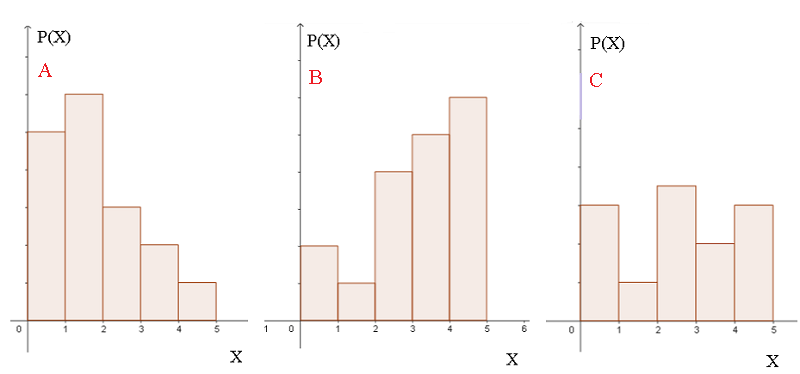Figure 1

Figure 2 shows a symmetric probability histogram whose data is concentrated in the middle. The mean $$\mu$$ and the median of this data are very close and approximately equal to $$3.5$$.

$$\mu \approx 3.5$$ , median $$\approx 3.5$$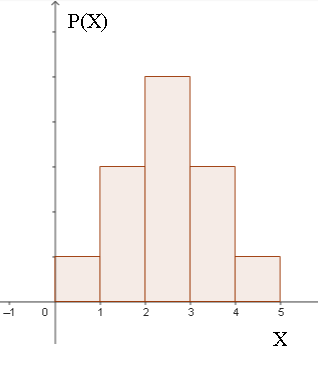Figure 2

The distribution is symmetric and will be discussed in more detail below.

## Normally Distributed Data

The probability histogram of a data set of 2560 data values in a Google sheet data file (1) is shown below. The data in this file may be downloaded and used for more practice.
Calculations, done using Google sheets, of the mean, median, and standard deviation of this data is shown below. Rounded to the nearest tenth, the mean of this data set $$\mu$$ is equal to $$3.5$$ and its standard deviation stdev is equal to $$1$$. Note also that the mean and the median are very close.Figure 3Figure 4

In figure 5 below, we have the probability histogram and the graph of the function $$f_{X}(x)$$ given by $f_{X}(x) = \dfrac{1}{\sqrt{2 \; \pi }} \; e^{-\frac{1}{2} (x-3.5)^2 }$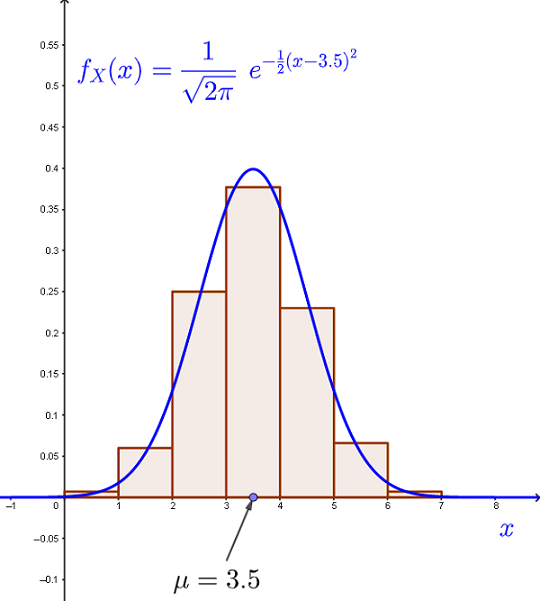Figure 5

We now use numerical values to explain the link between the area under the rectangles making the histogram and the area between the curve of the function $$f_X(x) = \dfrac{1}{\sqrt{2 \; \pi }} \; e^{-\frac{1}{2} (x-3.5)^2 }$$ and the x-axis.

Let $$P( 3 \le X \le 5)$$ be the probability that a data value $$X$$, selected randomly from the data set, is greater than or equal to $$3$$ and less than or equal to $$5$$. According to the classes [3-4] and [4-5] and their corresponding probabilities in Figure 4, we have
$$\qquad P( 3 \le X \le 5) \approx 0.377+0.229 = 0.606 \qquad (A)$$

We now use the
probability density function $$f_{X}(x)$$. The area between the curve of $$f_{X}(x)$$, the x-axis and $$x = 3$$ and $$x = 5$$ is given by:
$$\displaystyle \qquad P( 3 \le X \le 5) = \int_3^5 \; f_{X} (x) \; dx$$

Substitute $$f_{X}(x)$$ by $$\dfrac{1}{\sqrt{2 \; \pi }} \; e^{-\frac{1}{2} (x-3.5)^2 }$$
$$\displaystyle \qquad P( 3 \le X \le 5) = \dfrac{1}{\sqrt{2 \; \pi }} \int_3^5 \; \; e^{-\frac{1}{2} (x-3.5)^2 } \; dx$$
Use a calculator to obtain
$$\displaystyle \qquad P( 3 \le X \le 5) \approx 0.62465 \qquad (B)$$

Comparing the results of the probabilities in $$(A)$$ and $$(B)$$ found above, we conclude that it is possible to define $$f_{X}(x)$$ as the probability density function whose area may be used to determine probabilities.

Function $f_X(x) = \dfrac{1}{\sqrt{2 \; \pi }} \; e^{-\frac{1}{2} (x-3.5)^2 }$ is defined as a normal distribution with mean $$\mu = 3.5$$ and standard deviation $$\sigma = 1$$

In what follows, we will generalize the definition of a normal distribution and its properties.

## Probability Density Function of a Normal Distribution

The probability density function of a normal distribution with mean $$\mu$$ and standard deviation $$\sigma$$ is defined by $\boxed f_X(x) = \dfrac{1}{ \sigma \sqrt{2 \; \pi }} \; e^{-\frac{1}{2} \left( \dfrac{x - \mu}{\sigma} \right)^2 } }$ where $$X$$ is the normally distributed random variable.

### Graphs of Density Function of a Normal Distribution

In figure 6 below, are shown normal probability density functions with the same standard deviation $$\sigma = 2$$ and different means.Figure 6

In figure 7 below, are shown normal probability density functions with the same mean $$\mu = 4$$ and different standard deviations $$\sigma$$.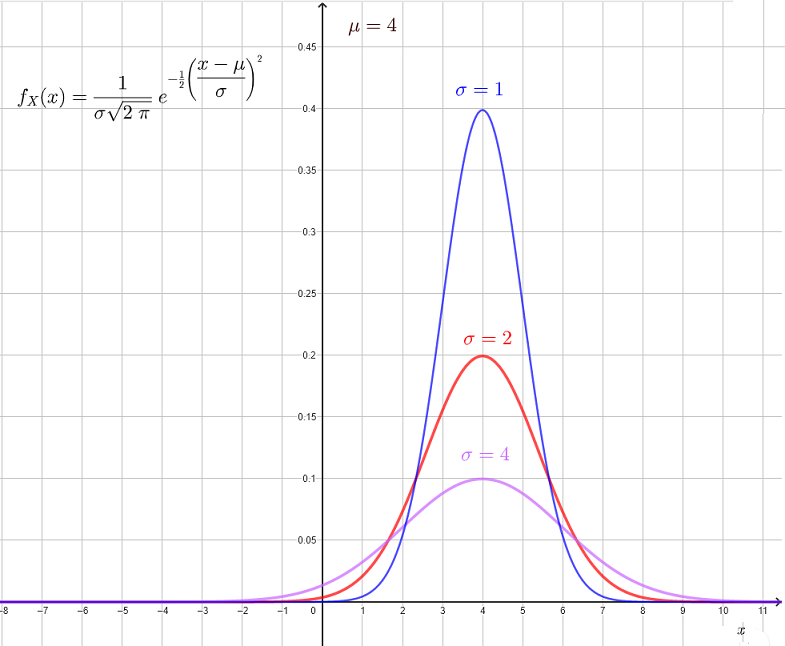Figure 7

From the above, the mean $$\mu$$ indicates the horizontal shifting of $$f_X(x)$$ and $$\sigma$$ indicates how data is grouped around the mean. For highly concentrated data, around the mean, $$\sigma$$ is small.

### Properties of Normal Distributions

1-The normal distribution is centered around the mean; this is clearly shown in figures 6 and 7.
2 - The mean and median of a normal distribution are very close (equal in theory).
3 - The total area under the curve of a normal distribution is equal to $$1$$. $\displaystyle \dfrac{1}{ \sigma \sqrt{2 \; \pi }} \int_{-\infty}^{\infty} \; e^{-\frac{1}{2} \left( \dfrac{x - \mu}{\sigma} \right)^2 } dx = 1$ 4 - Data Distribution is as follows
a) Approximately, $$68\%$$ of the data lies within 1 standard deviation from the mean. $\displaystyle \frac{1}{\sigma\sqrt{2\pi}} \int _{\mu-\sigma}^{\mu+\sigma}\:e^{-0.5\left(\frac{x-mu}{\sigma}\right)^2}dx \approx 0.68268$Figure 8

b) Approximately, $$95\%$$ of the data lies within 2 standard deviations from the mean.. $\frac{1}{\sigma\sqrt{2\pi \:}}\: \int _{\mu-2\sigma}^{\mu+2\sigma}\:e^{-0.5\left(\frac{x-mu}{\sigma}\right)^2}dx \approx 0.95449$Figure 9

c) Approximately, $$99\%$$ of the data lies within 3 standard deviations from the mean.. $\frac{1}{\sigma\sqrt{2\pi \:}}\: \int _{\mu-3\sigma}^{\mu+3\sigma}\:e^{-0.5\left(\frac{x-mu}{\sigma}\right)^2}dx \approx 0.99730$Figure 10

## Standard Normal Distribution and its Cumulative Probability

The normal distribution with mean $$\mu = 0$$ and standard deviation $$\sigma = 1$$ is called standard normal distribution and its probability density function is given by $f_X(x) = \dfrac{1}{ \sqrt{2 \; \pi }} \; e^{-\frac{1}{2} x^2 }$ The cumulative probability distribution of the standard normal distribution is defined by $\displaystyle F_{X} (x) = \dfrac{1}{ \sqrt{2 \; \pi }} \int_{-\infty}^{x} \; e^{-\frac{1}{2} t^2} \; dt$ and is used to find probabilities of the form $P( X \le a) = F_{X} (a)$Figure 11

Hence $$P( X \le a)$$ is given by the area between x-axis, the curve of the standard normal distribution and $$x = a$$
The integral defining the cumulative probability of the standard normal distribution is not given in a closed form and therefore can either be done using a
normal probability calculator or tables and may be downloaded for personal use. as in Google sheets shown below.

The cumulative probability may also be calculated using Google sheets and the function "=NORM.S.DIST(a)" as shown below.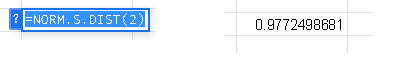Figure 12

## From Normal Distribution to Standard Normal Distribution

The normal distribution of mean $$\mu$$ and standard deviation $$\sigma$$ is given by $\displaystyle f_{X}(x) = \frac{1}{\sigma\sqrt{2\pi \:}}\:e^{-\frac{1}{2}\left(\frac{x-\mu}{\sigma}\right)^2}$ The probability $$P( X \le a)$$ is given by the area between the x-axis, the curve of the normal distribution and $$x = a$$ and is given by $\displaystyle P( X \le a) = F_{X} (a) = \frac{1}{\sigma \sqrt{2\pi \:}}\:\int_{-\infty}^{a} \; e^{- \frac{1}{2} \left(\frac{t - \mu}{\sigma}\right)^2} \; dt$ Let us use the substitution in the integral $$z = \frac{t - \mu}{\sigma}$$ which gives $$\dfrac{dz}{dt} = \dfrac{1}{\sigma}$$ and substitute in the above integral $\displaystyle P( X \le a) = F_{X} (a) = \frac{1}{\sqrt{2\pi \:}}\:\int_{-\infty}^{\frac{a - \mu}{\sigma}} \; e^{- \frac{1}{2} z^2} \; dz$ We are now dealing with the integral of the standard normal distribution and z-score given by. $z = \frac{a - \mu}{\sigma}$
The above result tells us that you only need to know the integral of the standard normal distribution in order to calculate any probability related to any normal distribution and that is by using the z-score defined above.
The integral $\frac{1}{\sqrt{2\pi \:}}\:\int_{-\infty}^{z_0} \; e^{- \frac{1}{2} z^2} \; dz$ can be calculated using Google sheets that may be downloaded for personal use. and is also given in the form of Table of Normal Distribution in pdf may be downloaded and used.

## Examples of Probabilities Related to Normal Distributions

Example 1
A random variable $$X$$ is normally distibuted with a mean $$\mu = 2.2$$ and a standard deviation $$\sigma = 2.5$$. Find the probability
a) $$\qquad P( X \le 1.2)$$
Solution to Example 1

In this example we have $$\mu = 2.2$$ and $$\sigma = 2.5$$, hence the z-score defined above is given by $z = \frac{1.2 - 2.2}{2.5} \approx -0.4$ Different ways to calculate the integral
a) Using a calculator, the probability is given by $P( X \le 1.2) = \frac{1}{\sqrt{2\pi \:}}\:\int_{-\infty}^{-0.4} \; e^{- \frac{1}{2} z^2} \; dz \approx 0.34457$ b) Use a table of values of the probability of a standard normal distribution which may beFigure 13

NOTE a Table of Normal Distribution in pdf may be downloaded and used.

Example 2
A random variable $$X$$ is normally distibuted with a mean $$\mu = -2.5$$ and a standard deviation $$\sigma = 2$$. Find the following probabilities
a) $$\qquad P( 0.5 \le X \le 3.1)$$
b) $$\qquad P( X \ge 0.8)$$
Solution to Example 2
a)
$$P( 0.5 \le X \le 3.1)$$ is the area between the x-axis, the curve of the normal distribution mean $$\mu = -2.5$$ and a standard deviation $$\sigma = 2$$ and $$x = 0.5$$ and $$x = 3.1$$
Hence
$$P( 0.5 \le X \le 3.1) = P( X \le 3.1) - P( X \le 0.5)$$

Let $$z_1 = \dfrac{0.5 - (-2.5)}{2} = 1.5$$ and $$z_2 = \dfrac{3.1 - (-2.5)}{2} = 2.8$$

We write the probabilities using the
z-score and use the table Table of Normal Distribution to obtain:
$$P( X \le 3.1) = P( Z_1 \le 2.8) = 0.9974448697$$
$$P( X \le 0.5) = P( Z_2 \le 1.5) = 0.9331927987$$
$$P( 0.5 \le X \le 3.1) = 0.9974448697 - 0.9331927987 = 0.064252071$$

NOTE that you can also use the Normal Probability Calculator to check the answer.
b)
$$P( X \ge 0.8) = 1 - P( X \le 0.8)$$
Let $$z_3 = \dfrac{0.8 - (-2.5)}{2} = 1.65$$

We write the probabilities using the
z-score and use the table Table of Normal Distribution to obtain:
$$P( X \le 0.8) = P( Z_3 \le 1.65) = 0.950528532$$
$$P( X \ge 0.8) = 1 - 0.950528532 = 0.049471468$$

NOTE that you can also use the Normal Probability Calculator to check the answer.
More
normal distribution problems with solutions are included in this site.